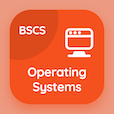Computer Science Courses Online

Digital Logic Design Practice Tests

Digital Logic Design Tests

The Book Half Subtractors Multiple Choice Questions (MCQ Quiz) PDF, Half Subtractors MCQs download to practice online digital logic design tests. Practice Combinational Logics Multiple Choice Questions and Answers (MCQs), Half Subtractors quiz answers PDF for top computer science schools. The eBook Half Subtractors MCQ App Download: subtractors in combinational logics, half adders, design procedure in combinational logics, multi level nor circuits test prep for associates in computer science.

The MCQ: Half subtractor is used to perform subtraction of PDF, "Half Subtractors MCQ" App Download (Free) with 2 bits, 3 bits, 4 bits, and 5 bits choices for top computer science schools. Study half subtractors quiz questions, download Google eBook (Free Sample) for online degrees.

## Logic Design: Half Subtractors MCQs

MCQ: Half subtractor is used to perform subtraction of

A) 2 bits
B) 3 bits
C) 4 bits
D) 5 bits

MCQ: How many outputs are required for the implementation of a subtractor?

A) 4
B) 3
C) 2
D) 1

MCQ: Two bit subtraction is done by

A) DE multiplexer
B) Multiplexer
C) full subtract or
D) half subtract or

MCQ: The simplified expression of half subtractor borrow is

A) B=x+y
B) B=xy
C) B=x'y
D) B=xy'

MCQ: The result of two bit subtraction is known as

A) difference bit
B) least significant bit
C) most significant bit
D) carry bit

### Practice Tests: Digital Logic Design Exam Prep

Download Digital Logic Design Quiz App, DBMS MCQ App, and Operating Systems MCQs App to install for Android & iOS devices. These Apps include complete analytics of real time attempts with interactive assessments. Download Play Store & App Store Apps & Enjoy 100% functionality with subscriptions!